# Nonlinear pricing Second degree price discrimination ECO 171

• Slides: 24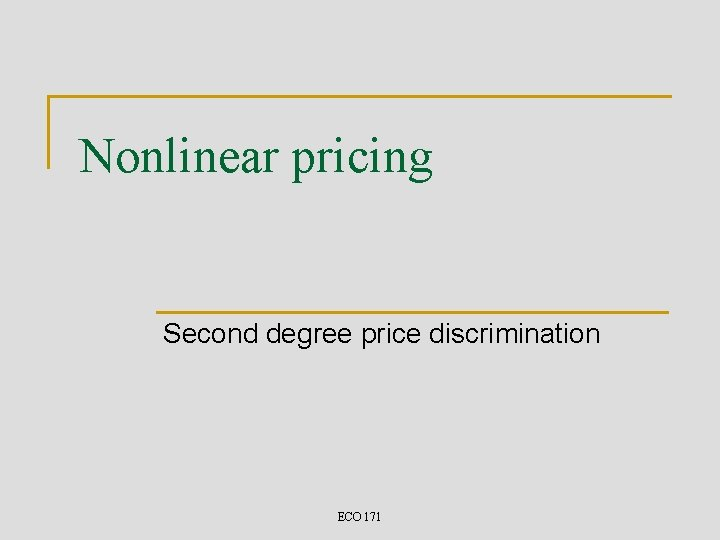Nonlinear pricing Second degree price discrimination ECO 171Self-selection n What if the seller cannot distinguish between buyers? q n n Then the type of price discrimination just discussed is impossible High-income buyer will pretend to be a low-income buyer q q n perhaps they differ in income (unobservable) to avoid the high entry price to pay the smaller total charge Take a specific example q q q Ph = 16 – Qh Pl = 12 – Ql MC = 4 ECO 171Second-degree price discrimination 2 n First-degree price discrimination requires: q q n This will not work q q n High Income: entry fee \$72 and \$4 per drink or entry plus 12 drinks for a total charge of \$120 Low Income: entry fee \$32 and \$4 per drink or entry plus 8 drinks for total charge of \$64 high income types get no consumer surplus from the package designed for them but get consumer surplus from the other package so they will pretend to be low income even if this limits the number of drinks they can buy Need to design a “menu” of offerings targeted at the two types ECO 171Second-degree price discrimination 3 n n The seller has to compromise Design a pricing scheme that makes buyers q q n n Essence of second-degree price discrimination It is “like” first-degree price discrimination q q n the seller knows that there are buyers of different types but the seller is not able to identify the different types A two-part tariff is ineffective q n reveal their true types self-select the quantity/price package designed for them allows deception by buyers Use quantity discounting ECO 171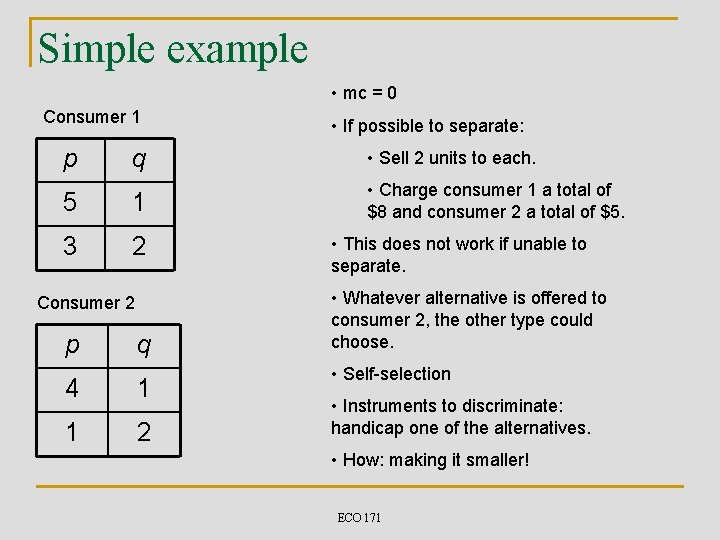Simple example • mc = 0 Consumer 1 • If possible to separate: p q • Sell 2 units to each. 5 1 • Charge consumer 1 a total of \$8 and consumer 2 a total of \$5. 3 2 Consumer 2 p q 4 1 1 2 • This does not work if unable to separate. • Whatever alternative is offered to consumer 2, the other type could choose. • Self-selection • Instruments to discriminate: handicap one of the alternatives. • How: making it smaller! ECO 171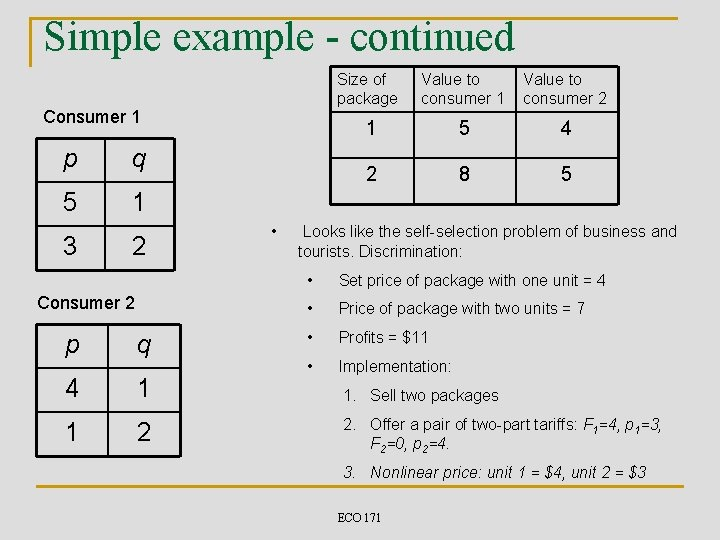Simple example - continued Consumer 1 p q 5 1 3 2 Consumer 2 p q 4 1 1 2 • Size of package Value to consumer 1 Value to consumer 2 1 5 4 2 8 5 Looks like the self-selection problem of business and tourists. Discrimination: • Set price of package with one unit = 4 • Price of package with two units = 7 • Profits = \$11 • Implementation: 1. Sell two packages 2. Offer a pair of two-part tariffs: F 1=4, p 1=3, F 2=0, p 2=4. 3. Nonlinear price: unit 1 = \$4, unit 2 = \$3 ECO 171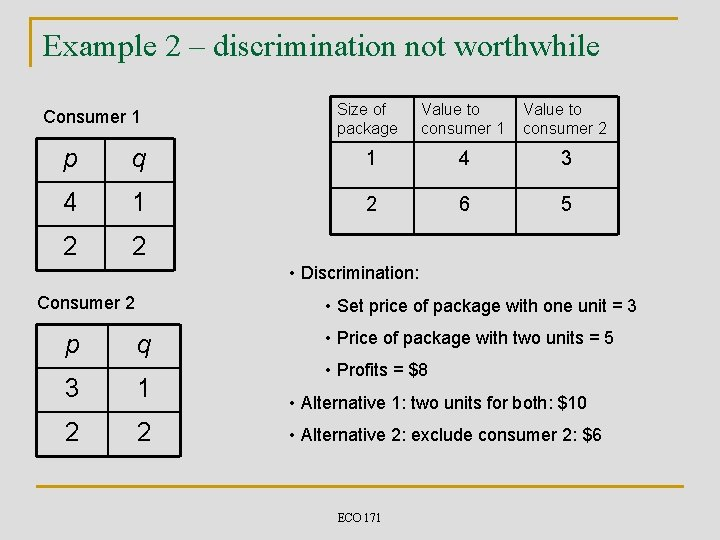Example 2 – discrimination not worthwhile Consumer 1 Size of package Value to consumer 1 Value to consumer 2 p q 1 4 3 4 1 2 6 5 2 2 • Discrimination: Consumer 2 p • Set price of package with one unit = 3 q 3 1 2 2 • Price of package with two units = 5 • Profits = \$8 • Alternative 1: two units for both: \$10 • Alternative 2: exclude consumer 2: \$6 ECO 171Example 2 – Exclude consumer 2 Consumer 1 Size of package Value to consumer 1 Value to consumer 2 p q 1 5 2 5 1 2 8 3 3 2 • Discrimination: Consumer 2 p • Set price of package with one unit = 2 q 2 1 1 2 • Price of package with two units = 5 • Profits = \$7 • Alternative 1: two units for both: \$6 • Alternative 2: exclude consumer 2: \$8 ECO 171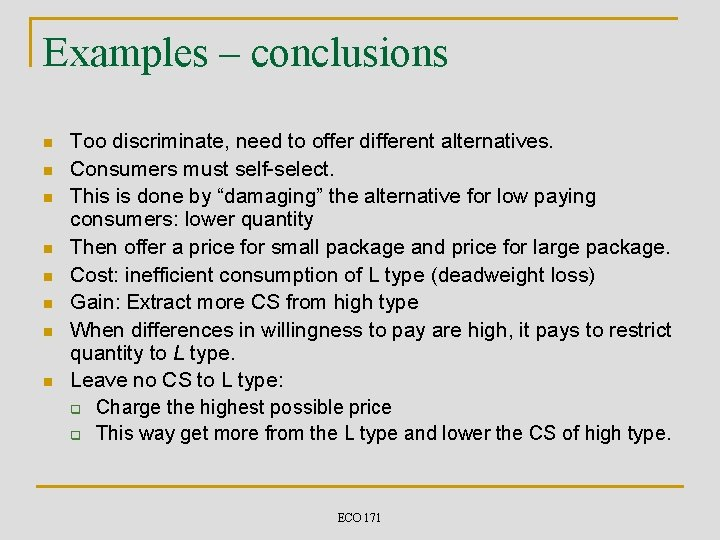Examples – conclusions n n n n Too discriminate, need to offer different alternatives. Consumers must self-select. This is done by “damaging” the alternative for low paying consumers: lower quantity Then offer a price for small package and price for large package. Cost: inefficient consumption of L type (deadweight loss) Gain: Extract more CS from high type When differences in willingness to pay are high, it pays to restrict quantity to L type. Leave no CS to L type: q Charge the highest possible price q This way get more from the L type and lower the CS of high type. ECO 171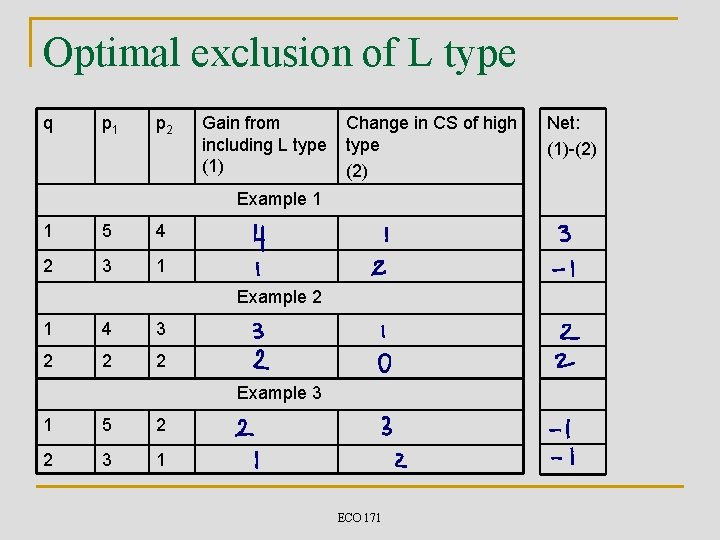Optimal exclusion of L type q p 1 p 2 Gain from including L type (1) Change in CS of high type (2) Example 1 1 5 4 2 3 1 Example 2 1 4 3 2 2 2 Example 3 1 5 2 2 3 1 ECO 171 Net: (1)-(2)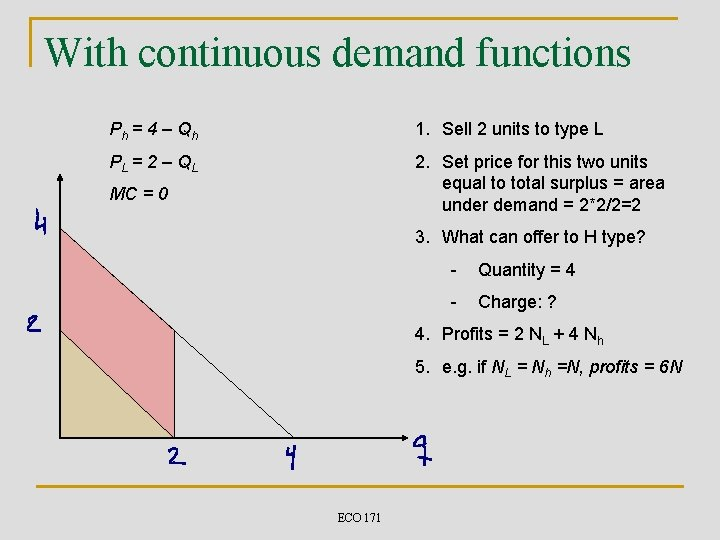With continuous demand functions Ph = 4 – Qh 1. Sell 2 units to type L PL = 2 – Q L 2. Set price for this two units equal to total surplus = area under demand = 2*2/2=2 MC = 0 3. What can offer to H type? - Quantity = 4 - Charge: ? 4. Profits = 2 NL + 4 Nh 5. e. g. if NL = Nh =N, profits = 6 N ECO 171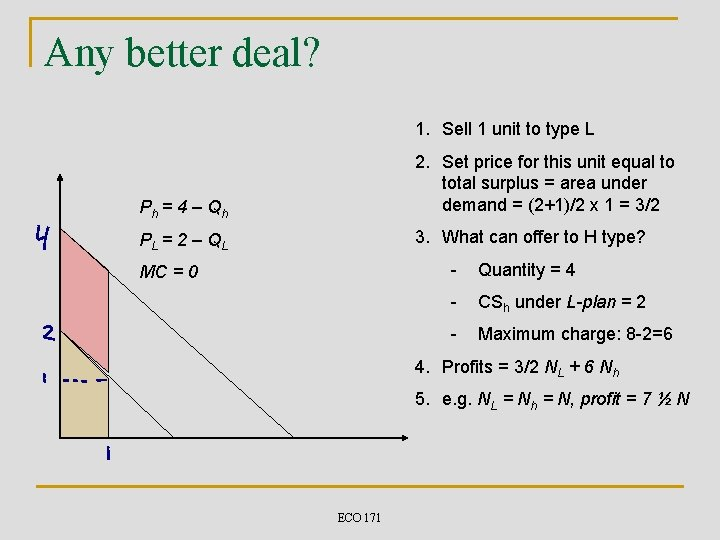Any better deal? 1. Sell 1 unit to type L Ph = 4 – Qh 2. Set price for this unit equal to total surplus = area under demand = (2+1)/2 x 1 = 3/2 PL = 2 – Q L 3. What can offer to H type? MC = 0 - Quantity = 4 - CSh under L-plan = 2 - Maximum charge: 8 -2=6 4. Profits = 3/2 NL + 6 Nh 5. e. g. NL = Nh = N, profit = 7 ½ N ECO 171Optimal quantity Effect of a small decrease in q. L -Loss from excluding L type: NL x Dq. L x p. L -D CSh = Nh x Dq. L x (ph – p. L ) - Net gain Dq. L [ Nh x (ph – p. L ) - NL x p. L ] If Nh=NL this is positive if ph-p. L>p. L. Optimal quantity q. L: cut quantity until Nh x (ph – p. L ) = NL x p. L Note: if marginal cost is positive, until Nh x (ph – p. L ) = NL x (p. L – mc ) Dq. L ECO 171Optimal quantity (continued) n n n In previous example, assuming Nh = NL ph = 4 -q, p. L= 2 -q Condition is: (4 -q – (2 -q)) = 2 -q q=0! Best is to exclude completely type L. Suppose NL = 2 x Nh q q n n 2 = 2 x (2 -q) q=1 Optimal quantity increases as NL / Nh increases Intuition: losses from excluding L are proportional to NL and gain from more surplus extraction from h are proportional to Nh. ECO 171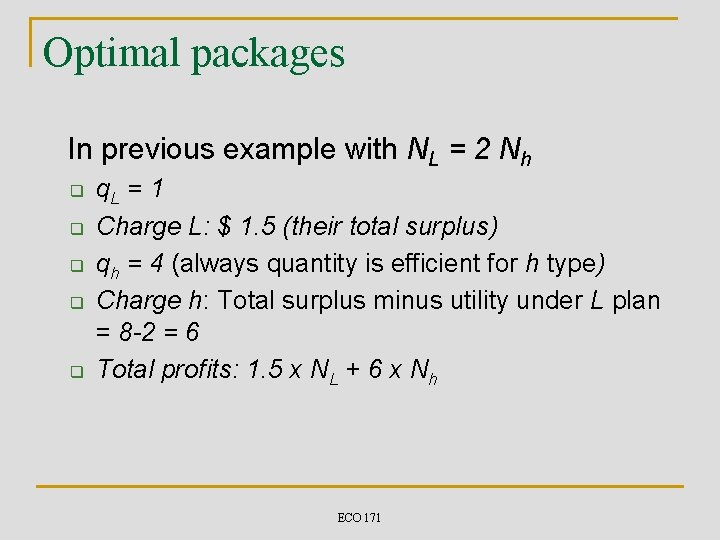Optimal packages In previous example with NL = 2 Nh q q q. L = 1 Charge L: \$ 1. 5 (their total surplus) qh = 4 (always quantity is efficient for h type) Charge h: Total surplus minus utility under L plan = 8 -2 = 6 Total profits: 1. 5 x NL + 6 x Nh ECO 171Optimal packages – general case q q Set q. L so that Nh x (ph-p. L) = NL x (p. L- mc) Charge L-type their entire surplus (area under demand curve up to q. L ) = Set qh at the efficient level: p(qh) = mc Charge H-type their entire surplus minus the utility they get under L-plan = ECO 171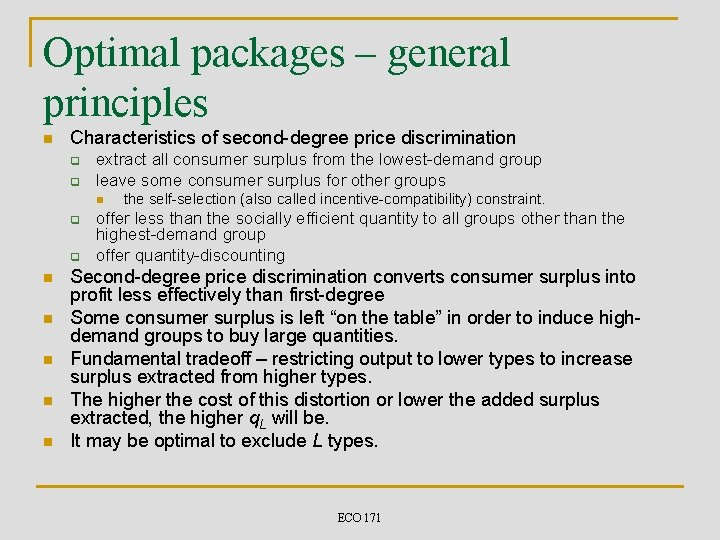Optimal packages – general principles n Characteristics of second-degree price discrimination q q extract all consumer surplus from the lowest-demand group leave some consumer surplus for other groups n q q n n n the self-selection (also called incentive-compatibility) constraint. offer less than the socially efficient quantity to all groups other than the highest-demand group offer quantity-discounting Second-degree price discrimination converts consumer surplus into profit less effectively than first-degree Some consumer surplus is left “on the table” in order to induce highdemand groups to buy large quantities. Fundamental tradeoff – restricting output to lower types to increase surplus extracted from higher types. The higher the cost of this distortion or lower the added surplus extracted, the higher q. L will be. It may be optimal to exclude L types. ECO 171Generalization to many consumer types Total price Optimal price schedule F 2 • Total price schedule is concave • Derivative interpreted as marginal price, i. e price for the last unit. • Can approximate by offering these two plans: F 1 T 1 (q) = F 1 + p 1 × q T 2 (q) = F 2 + p 2 × q Total quantity ECO 171Non-linear pricing and welfare n n Non-linear price discrimination raises profit Does it increase social welfare? q q Price suppose that inverse demand of consumer group i is P = Pi(Q) marginal cost is constant at MC – c suppose quantity offered to consumer group i is Qi total surplus – consumer surplus profit –is the area between the inverse demand marginal cost up to quantity Qi ECO 171 Demand Total Surplus c MC Qi Qi(c) Quantity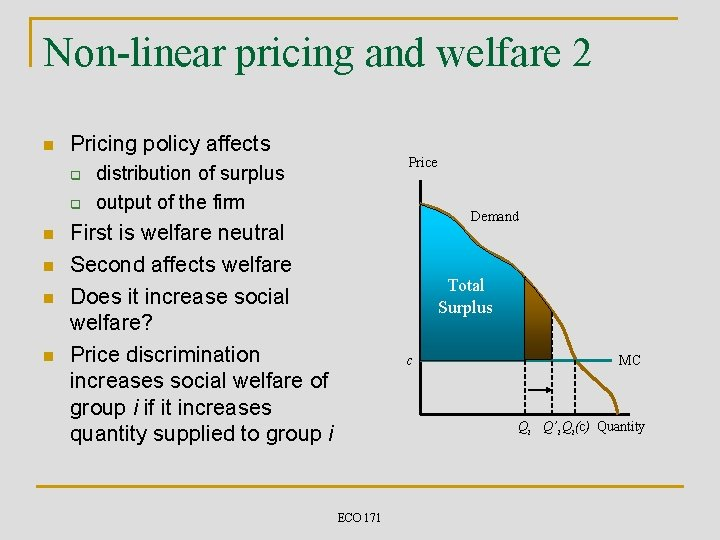Non-linear pricing and welfare 2 n Pricing policy affects q q n n Price distribution of surplus output of the firm Demand First is welfare neutral Second affects welfare Does it increase social welfare? Price discrimination increases social welfare of group i if it increases quantity supplied to group i Total Surplus c MC Qi Q’i Qi(c) Quantity ECO 171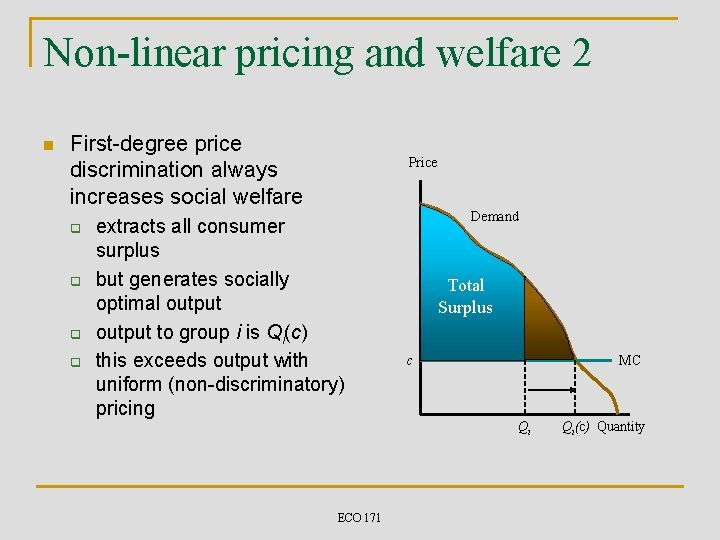Non-linear pricing and welfare 2 n First-degree price discrimination always increases social welfare q q Price extracts all consumer surplus but generates socially optimal output to group i is Qi(c) this exceeds output with uniform (non-discriminatory) pricing ECO 171 Demand Total Surplus c MC Qi Qi(c) QuantityNon-linear pricing and welfare 3 n Menu pricing is less straightforward q suppose that there are two markets n n • • Price low demand high demand PU Uniform price is PU Menu pricing gives quantities Q 1 s, Q 2 s Welfare loss is greater than L Welfare gain is less than G Low demand offered less than the socially optimal quantity L MC Qls Ql. U Quantity Price High demand offered the socially optimal quantity PU G Qh. U Qhs ECO 171 MC Quantity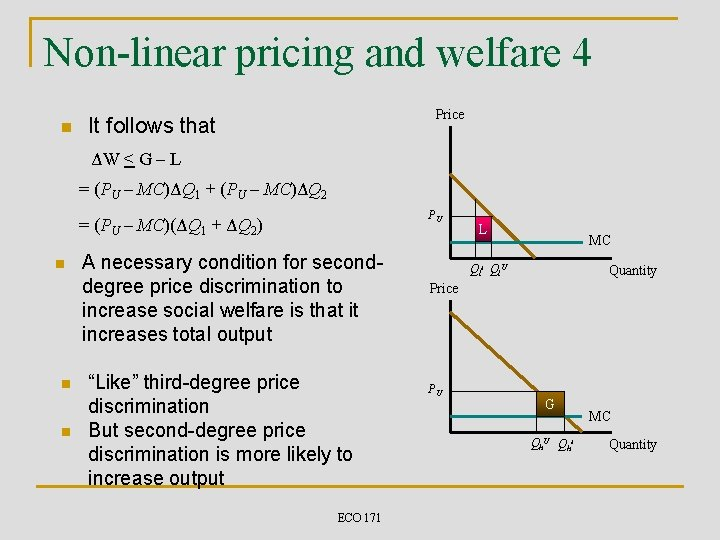Non-linear pricing and welfare 4 n Price It follows that ΔW < G – L = (PU – MC)ΔQ 1 + (PU – MC)ΔQ 2 PU = (PU – MC)(ΔQ 1 + ΔQ 2) n n n A necessary condition for seconddegree price discrimination to increase social welfare is that it increases total output “Like” third-degree price discrimination But second-degree price discrimination is more likely to increase output ECO 171 L MC Qls Ql. U Quantity Price PU G Qh. U Qhs MC Quantity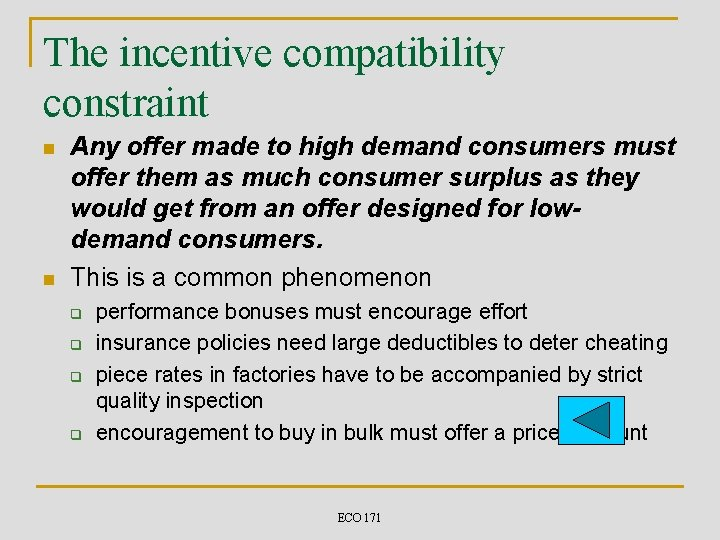The incentive compatibility constraint n n Any offer made to high demand consumers must offer them as much consumer surplus as they would get from an offer designed for lowdemand consumers. This is a common phenomenon q q performance bonuses must encourage effort insurance policies need large deductibles to deter cheating piece rates in factories have to be accompanied by strict quality inspection encouragement to buy in bulk must offer a price discount ECO 171